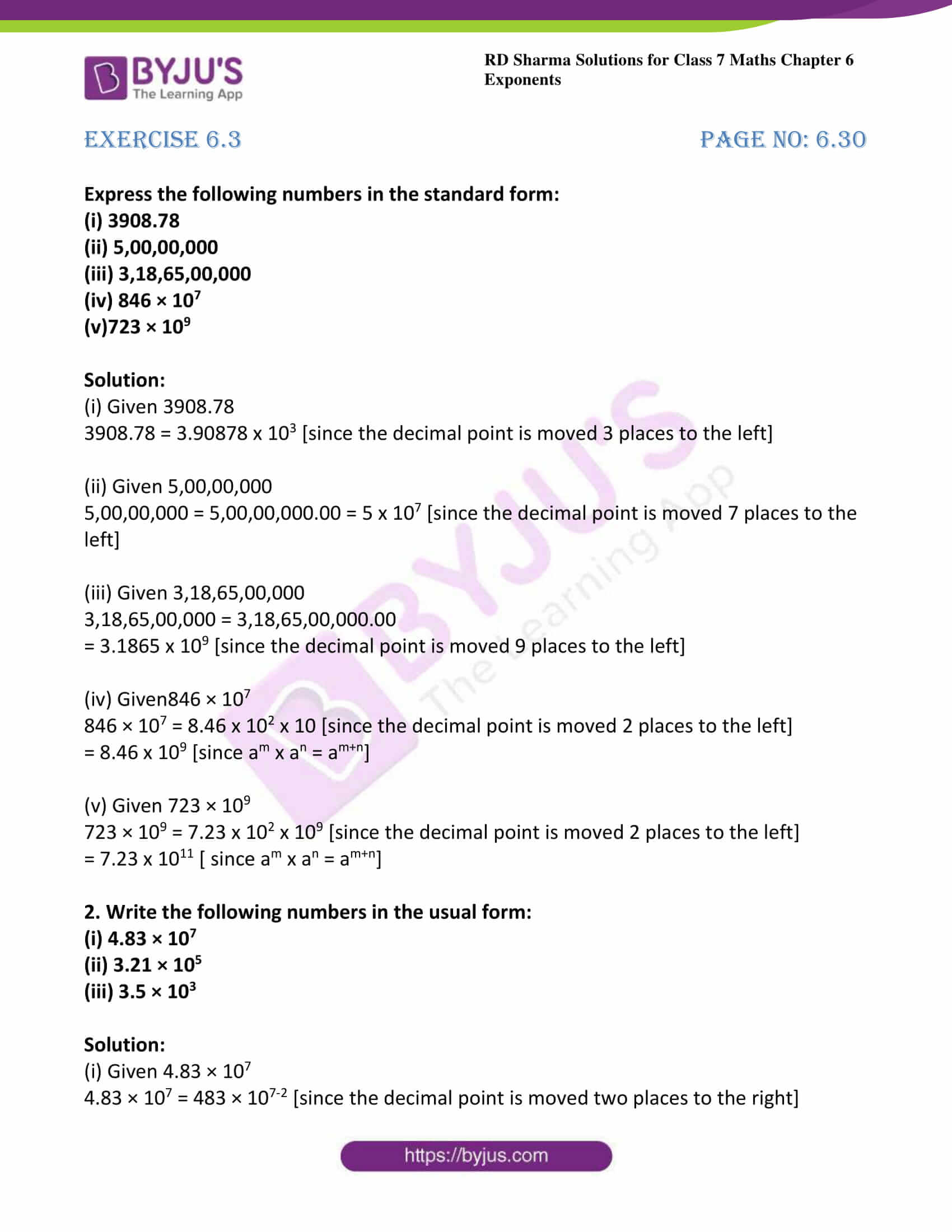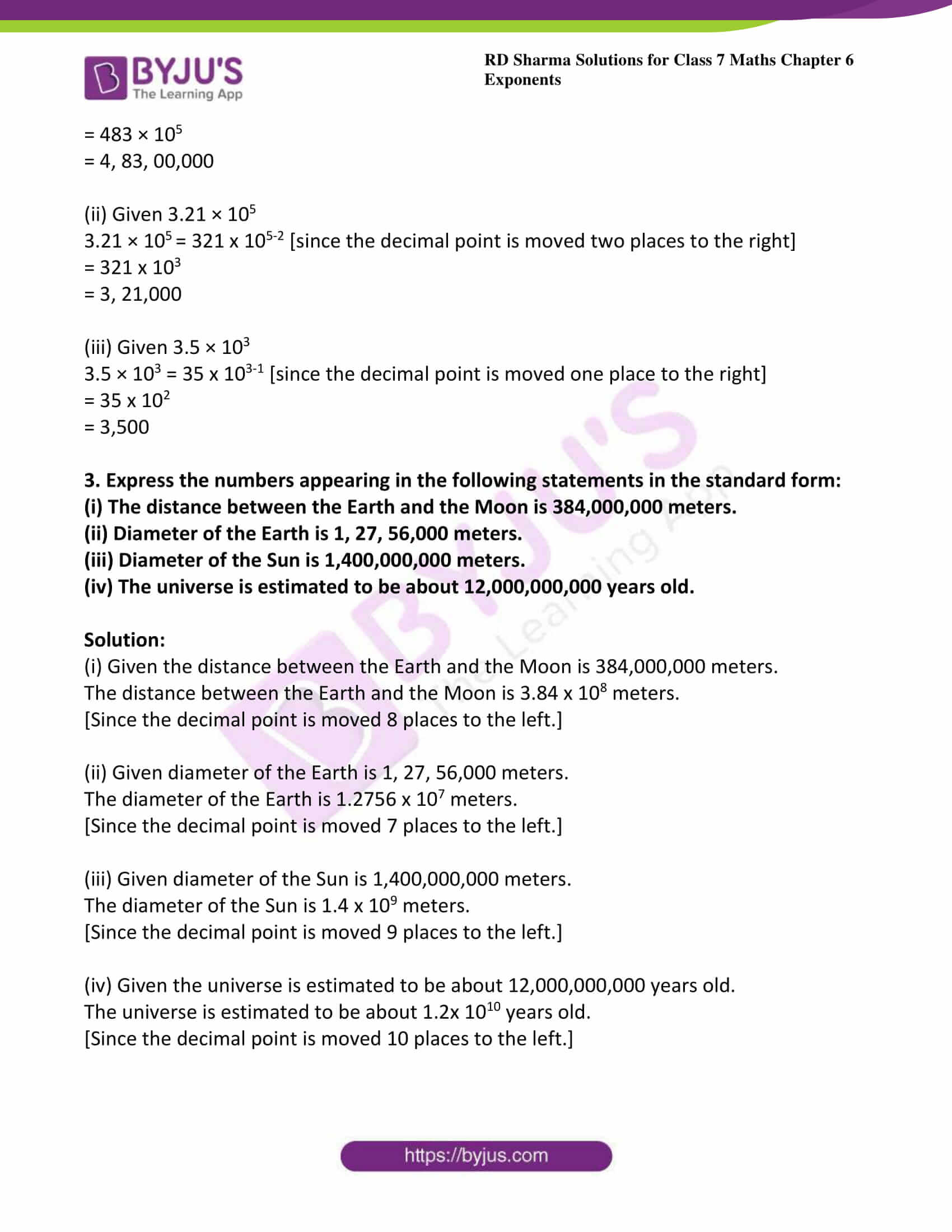# RD Sharma Solutions For Class 7 Maths Exercise 6.3 Chapter 6 Exponents

The solutions of RD Sharma for Class 7 Maths Exercise 6.3 of Chapter 6 Exponents are available in simple PDF format, which can be easily downloaded by the students from the provided links. This exercise formulated by BYJU’S experts, and it can be used as a model of reference by the students to improve their conceptual knowledge. In order to excel in exams, we suggest students go through RD Sharma Solutions for Class 7 Maths. Exercise 6.3 of class 7 helps the students to understand the concept of a standard form. A number is said to be in a standard form if it is expressed as the product of a number between 1 and 10 and a positive integer power of 10. It also deals with the use of exponents in expressing large numbers in standard form.

## Download the PDF of RD Sharma Solutions For Class 7 Maths Chapter 6 – Exponents Exercise 6.3### Access answers to Maths RD Sharma Solutions For Class 7 Chapter 6 – Exponents Exercise 6.3

Express the following numbers in the standard form:
(i) 3908.78
(ii) 5,00,00,000
(iii) 3,18,65,00,000
(iv) 846 × 107
(v)723 × 109

Solution:

(i) Given 3908.78

3908.78 = 3.90878 x 103 [since the decimal point is moved 3 places to the left]

(ii) Given 5,00,00,000

5,00,00,000 = 5,00,00,000.00 = 5 x 107 [since the decimal point is moved 7 places to the left]

(iii) Given 3,18,65,00,000

3,18,65,00,000 = 3,18,65,00,000.00

= 3.1865 x 109 [since the decimal point is moved 9 places to the left]

(iv) Given846 × 107

846 × 107 = 8.46 x 102 x 10 [since the decimal point is moved 2 places to the left]

= 8.46 x 109 [since am x an = am+n]

(v) Given 723 × 109

723 × 109 = 7.23 x 102 x 109 [since the decimal point is moved 2 places to the left]

= 7.23 x 1011 [ since am x an = am+n]

2. Write the following numbers in the usual form:
(i) 4.83 × 107
(ii) 3.21 × 105
(iii) 3.5 × 103

Solution:

(i) Given 4.83 × 107

4.83 × 107 = 483 × 107-2 [since the decimal point is moved two places to the right]

= 483 × 105

= 4, 83, 00,000

(ii) Given 3.21 × 10

3.21 × 10= 321 x 105-2 [since the decimal point is moved two places to the right]

= 321 x 103

= 3, 21,000

(iii) Given 3.5 × 103

3.5 × 103 = 35 x 103-1 [since the decimal point is moved one place to the right]

= 35 x 102

= 3,500

3. Express the numbers appearing in the following statements in the standard form:

(i) The distance between the Earth and the Moon is 384,000,000 meters.
(ii) Diameter of the Earth is 1, 27, 56,000 meters.
(iii) Diameter of the Sun is 1,400,000,000 meters.
(iv) The universe is estimated to be about 12,000,000,000 years old.

Solution:

(i) Given the distance between the Earth and the Moon is 384,000,000 meters.

The distance between the Earth and the Moon is 3.84 x 108 meters.

[Since the decimal point is moved 8 places to the left.]

(ii) Given diameter of the Earth is 1, 27, 56,000 meters.

The diameter of the Earth is 1.2756 x 107 meters.

[Since the decimal point is moved 7 places to the left.]

(iii) Given diameter of the Sun is 1,400,000,000 meters.

The diameter of the Sun is 1.4 x 109 meters.

[Since the decimal point is moved 9 places to the left.]

(iv) Given the universe is estimated to be about 12,000,000,000 years old.

The universe is estimated to be about 1.2x 1010 years old.

[Since the decimal point is moved 10 places to the left.]